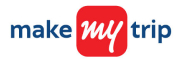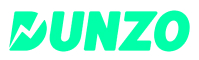New update is available. Click here to update.
Last Updated: 8 Jul, 2020

# Product Of Array Except Self

Easy+24 more companies

## Problem statement#### You have been given an integer array/list (ARR) of size N. You have to return an array/list PRODUCT such that PRODUCT[i] is equal to the product of all the elements of ARR except ARR[i]

##### Note :
``````Each product can cross the integer limits, so we should take modulo of the operation.

Take MOD = 10^9 + 7 to always stay in the limits.
``````
``````Can you try solving the problem in O(1) space?
``````
##### Input format :
``````The first line contains an integer 'T' which denotes the number of test cases or queries to be run. Then the test cases follow.

The first line of each test case or query contains an integer 'N' representing the size of the array/list.

The second line contains 'N' single space-separated integers representing the elements in the array/list.
``````
##### Output Format :
``````For each test case, print the elements of the 'PRODUCT' array separated by a single space.

Output for every test case will be printed in a separate line.
``````

#### Important Note :

``````You are required to return the product array and no need to print the result explicitly. It has already been taken care of.
``````
##### Constraints :
``````1 <= T <= 100
0 <= N <= 10^5
0 <= ARR[i] <= 10^5

Time Limit: 1 sec
``````## Approaches

### 01 ApproachTraverse through every index of the array/list and compute the product of all the other elements in the array/list. This would require a new array/list of the same size as that of the input array/list in which we will store the product of the elements corresponding to every index.

1. Create a new array/list to store the product at every index

2. Start traversing on the input array/list till its length

3. For every ith iteration, find the product of all the elements to the left of i [0 - (i - 1)] and the product of all the elements to the right of i [(i + 1)  - (n -1)]

4. Take the product of left and right and assign the result to the i-th position in the new array keeping the result for every i

5. Repeat the above two steps till the end of the input array

6. Return the array in which you stored the products